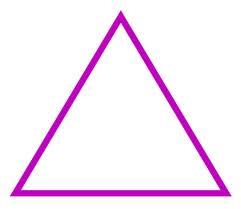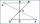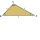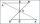# 3-bracket 2

May be the smallest angle in the triangle greater than 70°?

Result

#### Solution:

$70^\circ \ge \dfrac{ 180^\circ}{3} = 60^\circ$Our examples were largely sent or created by pupils and students themselves. Therefore, we would be pleased if you could send us any errors you found, spelling mistakes, or rephasing the example. Thank you!

Leave us a comment of this math problem and its solution (i.e. if it is still somewhat unclear...):Be the first to comment!Tips to related online calculators

#### You need to know the following knowledge to solve this word math problem:

We encourage you to watch this tutorial video on this math problem:

## Next similar math problems:

• TrianglesIvo wants to draw all the triangles whose two sides of which have a length of 4 cm and 9 cm and the length of the third side is expressed in whole centimeters. How many triangles does he have?
• Possible lengthsFind the most possible lengths for the third side of a triangle with sides 20 and 18.
• TrianglesFive sticks with a length of 2,3,4,5,6 cm. How many ways can you choose three sticks to form three sides of a triangle?
• TrianglesHanka cut the 20 cm long straws into three pieces each piece had a length in cm. Then, with these three pieces, she tried to make a triangle. a) What circuit has each of the triangles? b) How long can the longest side measure? c) How many different tria
• TriangleProve whether you can construct a triangle ABC, if a=9 cm, b=6 cm, c=10 cm.